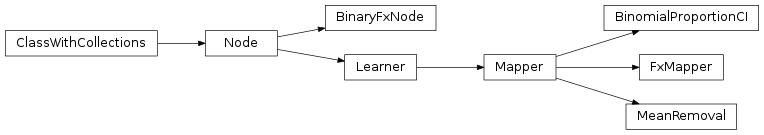# mvpa2.mappers.fx¶

Transform data by applying a function along samples or feature axis.Functions

 `absolute_features`() Returns a mapper that converts features into absolute values. `argsort`(seq[, reverse]) Return indices to get sequence sorted `maxofabs_sample`() Returns a mapper that finds max of absolute values of all samples. `mean_feature`([attrfx]) Returns a mapper that computes the mean feature of a dataset. `mean_group_feature`(attrs[, attrfx]) Returns a mapper that computes the mean features of unique feature groups. `mean_group_sample`(attrs[, attrfx]) Returns a mapper that computes the mean samples of unique sample groups. `mean_sample`([attrfx]) Returns a mapper that computes the mean sample of a dataset. `merge2first`(attrs) Compress a sequence by discard all but the first element `subtract_mean_feature`([attrfx]) Subtract mean of features across samples. `sum_sample`([attrfx]) Returns a mapper that computes the sum sample of a dataset. `sumofabs_sample`() Returns a mapper that returns the sum of absolute values of all samples.

Classes

 `BinaryFxNode`(fx, space, **kwargs) Extract a dataset attribute and call a function with it and the samples. `BinomialProportionCI`(**kwargs) Compute binomial proportion confidence intervals `FxMapper`(axis, fx[, fxargs, uattrs, attrfx, ...]) Apply a custom transformation to (groups of) samples or features. `MeanRemoval`([in_place]) Subtract sample mean from features.